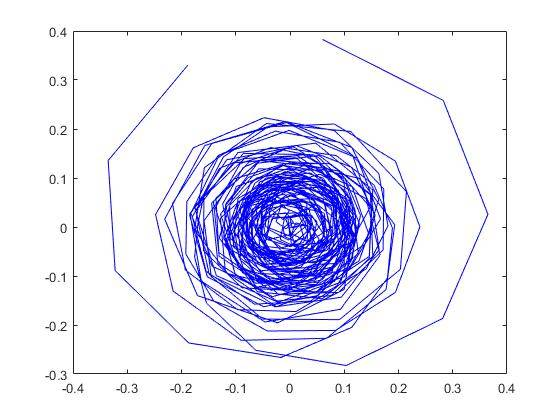# Question about Fourier transformation in Matlab

Hello everybody.

I am triying to calculate a band-pass filter using the fourier transform.
I have a vector with 660 compomponents; one for each month. I am looking for a phenomenon which has a periodicity between 3 and 7 years (it's el niño, on the souhtern pacific ocean). I want to make zero all the coefficients of the fourier transform except the ones which are in between 3 and 7 years. I have tried to remove all of the coefficients which are between the 36th (12*3) and 84th (12*7). Then, I proceed to do the same thing in the other half of the series to mantain simmetry.

But once I make the inverse fourier transform and I plot it, the results don't match whith what I want.

Can someone tell me where is my faliure?

Code used:
niniofourier=fft(ninios);

for i=1:35
niniofourierb(i)=0;
end

for i=85:330
niniofourierb(i)=0;
end

for i=36:84
niniofourierb(i)=niniofourier(i);
end

for i=331:576
niniofourierb(i)=0;
end

for i=577:624
niniofourierb(i)=niniofourier(i);
end

for i=625:660
niniofourierb(i)=0;
end

niniosi=ifft(niniofourierb);
figure(4)
plot (niniosi)

vela
Staff Emeritus
Homework Helper
You're selecting the wrong part of the DFT. You don't want coefficients 36 through 84.

You have 660 months worth of samples, so ##X_k## (for ##k=0, 1, \dots, 330##) corresponds to a cycle that has an angular frequency of ##k\omega_0## where ##\omega_0=2\pi/660\text{ rad/month}##. What you want to do is determine the range of ##k## that corresponds to angular frequencies between ##2\pi/36\text{ rad/month}## and ##2\pi/84\text{ rad/month}##.

•Frank Einstein
You're selecting the wrong part of the DFT. You don't want coefficients 36 through 84.

You have 660 months worth of samples, so ##X_k## (for ##k=0, 1, \dots, 330##) corresponds to a cycle that has an angular frequency of ##k\omega_0## where ##\omega_0=2\pi/660\text{ rad/month}##. What you want to do is determine the range of ##k## that corresponds to angular frequencies between ##2\pi/36\text{ rad/month}## and ##2\pi/84\text{ rad/month}##.

Thank you very much; I am using now this code:
for i=1:660
if (i-1)/660<pi/18
if (i-1)/660> pi/42
niniofourierb(i)=niniofourier(i);
else
niniofourierb(i)=0;
end
else
niniofourierb(i)=0;
end
end
niniosi=ifft(niniofourierb);
figure(4)
plot (niniosi)

But it seems like I still got it wrong. I am obtaining something which makes no senseI have done the same filter using a low pass filter and I obtain reasonalbe results.

vela
Staff Emeritus
Homework Helper
I have no idea what that plot is supposed to represent.

Don't forget that like before you also have to take care of the other half of the spectrum.

I have no idea what that plot is supposed to represent.

Don't forget that like before you also have to take care of the other half of the spectrum.

I have plotted the inverse fourier transform of the series in which all the coefficients except those between 2π/36 and 2π/84 are zero.
In the code that I have posted is figure(4)
EDIT:
auxTWO=fliplr(niniofourier(1:330));
for i=1:330
niniofourierb(i+330)=auxTWO(i);
end
niniosi=ifft(niniofourierb);
niniosib=niniosi(1:330);
If I add this code to make the mirroring of the first half, I still get an awful figure

Last edited:
FactChecker